# 1st PUC Chemistry Previous Year Question Paper March 2013 (South)

Students can Download 1st PUC Chemistry Previous Year Question Paper March 2013 (South), Karnataka 1st PUC Chemistry Model Question Papers with Answers helps you to revise the complete Karnataka State Board Syllabus and score more marks in your examinations.

## 1st PUC Chemistry Previous Year Question Paper March 2013 (South)

Time: 3.15 Hours
Max Marks: 90

Instructions:

1. Write the question number legibly in the margin.
2. Answer for a question should be continuous.

Part – A

I. Answer the EIGHT of the following: ( 8 × 1 = 8 )

Question 1.
What is functional group?
Properties of certain organic compounds determined by the presence of an atom or group of atom is called functional group.

Question 2.
Give one example which has Kc = Kp
H2(g) + I2(g) ⇌ 2NO(s)
or
N2 + O2 ⇌ 2NO(g).

Question 3.
State modern periodic law.
Properties of elements are the periodic function of their atomic number.Question 4.
Give any one example for solid and vapour equilibrium.
I2 (solid) ⇌ I2 (vapour)
OR
camphor (s) ⇌ camphor (vapour)

Question 5.
What is oxidation number of oxygen in hydrogen peroxide?
Oxygen in H2O2 = -1

Question 6.
Mention one use of hydrogen.
It is used as rocket fuel in space research.

Question 7.
Avogadro’s number = 6.022 × 1023

Question 8.
A wavelength of 100 nm of a radiation lies in which region?
100 nm = UV

Question 9.
State Boyle’s law.
At constant temperature the volume of given mass of a gas is inversely proportional to pressure.

Question 10.
Whether internal energy is extensive property or intensive property?
Extensive property.Question 11.
Write electronic configuration of alkali metals.
[Nobel gas] ns1.

Part – B

II. Answer any EIGHT of the following questions. ( 8 × 2 = 16 )

Question 12.
Calculate percentage composition of carbon and hydrogen in ethanol.
Molecular mass of ethanol = 46.06
% carbon = $$\frac{24}{46}$$ x 100 = 52
% of hydrogen = $$\frac{6}{46}$$ x 100 = 13

Question 13.
Explain hydrogen bonding.
Hydrogen bonding is defined as “Attractive force which binds hydrogen atom of one molecule with electronegative atom (F, O, or N) of another molecule.

Question 14.
Define Hund’s rule of maximum multiplicity.
Hund’s rule: “Pairing of electron in the orbitals having same subshell doesn’t take place until each orbital has one electron each”.

Question 15.
Represent the behaviour of real gases from ideal gases graphically.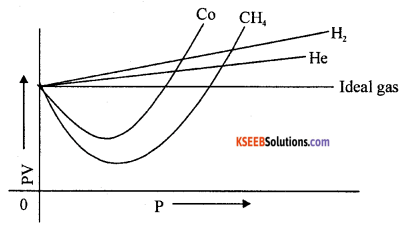Question 16.
Represent the chemical equation when butane (Cooking gas – LPG) burnt completely in air?
C4H10(g) + $$\frac{13}{2}$$ O2 (g) → 4CO2 (g) + 5H2O(g)   ∆cH = -2658.0 KJQuestion 17.
Explain lewis acid and base concept.
Acid → Accept a pair of electron
Base → Donate a pair of electron

Question 18.
Define ionisation enthalphy with an example.
Ionisation enthalpy “The amount of energy to remove outermost electron from an isolated gaseous atom”.
eg. Na – 498. 5 KJ/mol.

Question 19.
What is redox reaction? Give one example.
A chemical reaction in which both oxidation and reduction processes occur simultaneously are known as redox reaction.
eg. 2Na(s) + Cl2 (g) → 2NaCl(s)

Question 20.
Give any two uses of heavy water.

1. It is used as a mild bleaching agent.
2. Used an an antiseptic in surgery.

Question 21.
At equilibrium the concentration of N2 = 3.0 × 10-3 M, O2 = 4.2 × 10-3 M and NO = 2.8 × 10-3 M in a sealed tube. What will be Kc for the reaction N2(g)+ O2(g) ⇌ 2NO(g)?
Kc = $$\frac{\left[\mathrm{NO}_{2}\right]^{2}}{\left[\mathrm{O}_{2}\right]\left[\mathrm{N}_{2}\right]}$$
= $$\frac{2.8 \times 10^{-3}}{10^{-3} \times(3.0)(4.2) \times 10^{-3}}$$
= 0.622

Question 22.
What is resonance effect?
The permanent polarity is produced by the interaction of lone pair and n electrons in conjugate system.Question 23.
In sulphur estimation 0.157 g of organic compound gave 0.4813 g of barium sulphate calculate the percentage of sulphur in the compound.
Mol. mass of BaSO4 = 233
233 g of BaSO4 contain 32 g of sulphur
0.483 g of BaSO4 contain =
% Sulphur = $$\frac{32 \times 0.483 \times 100}{233 \times 0.157}$$ = 42.10%

Part – C

III. Answer any FOUR of the following questions : ( 4 × 4 = 16 )

Question 24.
(a) What is chromatography?
Chromatography: It is important technique which separates mixtures into their compounds and also tests the purity of compounds.

(b) Explain how is an organic compound separated by distillation method?
Distillation is method used to separate constituents of a liquid mixture which differ in their boiling points. Distillation is a process which involves two steps.

1. Vapourisation: Liquid is converted into vapours.
2. Condensation: Vapours are condensed again into liquid.

Question 25.
(a) Explain in brief how is nitrogen detected using sodium fusion extract?

 Experiment Observation Inference Organic compound + sodium fusion extract + boiled with FeSO4 + conc. H2SO4 Blue colour Compound is Nitrogen(b) Write the structure of 1,2 – Dibromobenzene.Question 26.
(a) Explain mechanism of chlorination of methane.
The mechanism involves three steps:
(1) Initiation : A chlorine molecule absorbs energy from sunlight to form free radicals.(2) Propagation : A chlorine free radical reacts with methane forming a HCl and methyl free radical.
CH4 + Cl → CH3+ HCl
(b) The methyl free radical attack chlorine molecule forming a methyl chloride and chlorine free radical
CH3+ Cl2 → CH3Cl + Cl
Termination : The two free radical combines to form product.
Cl + Cl → Cl (Chlorine)
CH3+CH3→ C2H6 (Ethane)
CH3+ Cl → CH3Cl (Methyl Chloride)

(b) Give one use of ethene.
Ethene is used for artificial ripening of fruits.Question 27.
(a) How is ethyne prepared from calcium carbide?
When calcium carbide react with water to form ethyne.
CaC2 + 2H2O → CH ≡ CH +Ca(OH)2

(b) Mention the formula of Huckle rule.
Huckel formula = (4n + 2) π electron

Question 28.
(a) Explain mechanism of nitration of benzene.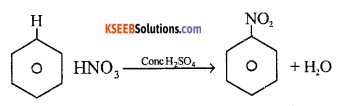Mechanism: Step 1: Generation of electrophile
OH – NO2 + H – HSO4 → NO2++ HSO4+ H2O
Step 2 : Attack of electrophile on benzene ring to form carbocation.Step 3 : The carbocation loses proton to form nitrobenzene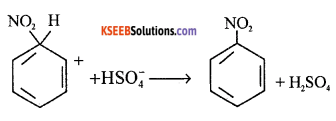(b) Which element is detected by kjeldhal method?
Nitrogen

Question 29.
(a) What is ozone hole? Explain.
One of the reason of ozone hole by a class of compounds called freons. Due to several kinds of human activities involving freons they will diffuse into ozone layer of the stratosphere.
They undergo photodissociation as follows: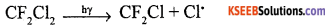The chlorine free radical react with ozone to form chlorine monoxide radical and O2
O3 + Cl → ClO + O2
ClO + O → O2 + Cl
The chlorine radicals are continuously regenerated causes damage of ozone layer. This is a ozone hole.

(b) Explain green house effect.
The heating of atmosphere due to absorption of IR radiation emitted by earth by the gases of atmosphere is called greenhouse effect.

Green house gases absorb large amount of energy from sunlight and transfer it as heat by which earth gets heated. But they do not readily radiate back the earth’s heat energy to the space. Instead they reflect back a part of the heat energy to the lower atmosphere.

Part – D

Answer any FIVE of the following questions : ( 5 × 5 = 25 )

Question 30.
(a) Why size of cation is smaller than its parent atom?
A cation is formed by the loss of electron which decrease in size due to more attraction by nucleus, because of increase in nuclear charge.

(b) Which of these isoelectronic species -Al3+ or Mg2+ has lower size?
Higher charges lower the size.
Al3+ has lower size than Mg2+.

(c) How is a chemical bond formed?
A bond is formed by the attraction force which holds various constituent together in different chemical species.Question 31.
(a) Define bond length.
The average distance between the centres of the nucleus of the two bonded atoms in a molecule.

(b) Explain sp3 hybridisation in methane.
The electronic configuation of carbon in the excited state is 1s1 2s1 2Px1  2Py1 2Pz1. One 2s orbital and 3p orbitals hybridise to give four hybrid orbitals. The hybrid orbitals are directed towards the comer of a regular tetrahedron. The angle between any two orbitals is 109°28′. The four sp3 hybrid orbitals of carbon atom overlap axially with s-orbitals of four hydrogen atom to give C – H σ bonds.
The C – H σ bonds is sp3 – s bond. H – C – H bond angle is 109°28′. The shape of the molecule is tetrahedral.Question 32.
(a) Write electronic configuration of lithium molecule.
11s)2 x1s)2 (σ2s)2

(b) Why Helium (He2) molecule does not exist.
Helium molecule has bond order equal to zero, hence molecule does not exist.

(c) Define bond order.
It is the number of covalent bonds holding the atoms in the molecule.

Question 33.
(a) Give any three postulates of molecular orbital theory.

1. The molecular orbitals are formed by the combination of atomic orbitals of comparable energies and proper symmetry.
2. The number of molecular orbitals formed is equal to the number of combining atomic orbitals.
3. The bonding molecular orbital has lower energy and hence greater stability than the corresponding antibonding molecular orbital.

(b) Differentiate between σ bond and π bond.

 Sigma bond Pi bond 1. Sigma bond formed by the axial combination of orbitals. 1. Pi bond formed by the head to head combination. 2. More efficient 2. Less efficient

Question 34.
(a) Mention rules used to balance a chemical reaction by oxidation number method.

1. Oxidation number of a mono atomic ion is same as the charge on it.
2. Oxidation number of metals are positive and those of non metals are negative.
3. In neutral compounds, the sum of oxidation number of all the atoms is equal to zero.
4. In case of polyatomic ion, the sum of the oxidation numbers of all the atoms of the ion is equal to charge on the ion.

(b) Give an example of covalent hydride.
CH4, NH3, HF, H2O

Question 35.
(a) How is temporary hardness of water removed?

1. By boiling of water.
2. By Clark’s method : In this method lime is added to the hard water. It precipitates out CaCO3 and Mg(OH)2 which can be filtered off.
Ca(HCO3) + Ca(OH)2 → 2CaCO3 ↓ +2H2O

(b) Give one biological importance of sodium and potassium ion.
Biological importance of sodium.
1. Transmit nerve signals.

Biological importance of potassium.
1. Activates many enzymes.

(c) Name the radioactive element of alkali metals.
Francium.Question 36.
(a) Potassium is lighter than sodium? Why?
Potassium has unusual increase of size (or) density of K = 0.86 g/cm3, density of Na = 0.97 g/cm3,
Hence potassium is lighter than sodium.

(b) Give any two uses of sodium carbonate.

1. Water softening
2. Laundering and cleaning.

(c) What happens when a piece of aluminium is added to dilute hydrochloric acid.
Aluminium dissolves in HCl to liberate hydrogen gas.
2Al + 6HCl → 2AlCl3 + 3H2

Question 37.
(a) How is borax prepared?
Borax can also be obtained by neutralisation of boric acid with Na2CO3
4H3BO3 + Na2CO3 → Na2B4O7 + 6H2O + CO2. ↑

(b) Write a short note on fullerene.
Fullerens are made by heating the graphite in an electric arc in the presence of inert gases. Fullerens are cage like molecules. It contains twenty six membered rings and twelve five membered rings. All the C-atoms are equal, they will undergo sp2 hybridisation. Each carbon atom form three sigma bonds with other three C-atoms. This ball shaped molecule has 60 vertices. It also contain both single and double bonds with C-C distances of 143.5 pm and 138.3 pm.

(c) Concentrated nitric acid can be transported in aluminium container. Give a reason.
Conc. HNO3 reacts with aluminium forms a protective layer called passive. It does not react further.

Part – E

Answer any FIVE of the following questions : ( 5 × 5 = 25 )

Question 38.
(a) Define Gay-Lussac’s law.
At constant volume, the pressure of a fixed mass of a gas is directly proportional to its temperature.

(b) What is SI unit of luminous intensity?
Unit – Candela or Cd.

(c) Define ‘mole’.
Mole : It is the amount of substance that contain as many particles (atoms, ions, molecules) exactly 12 g of the C12 isotopes.Question 39.
(a) What is meant by average atomic mass?
The susbtance which has isotopes, and found relative abundance.
eg 12C = 98.892
13C = 1.108
14C = 2 × 10-10(b) What is density? How is it calculated?
Density of a substance is the amount of mass per unit volume.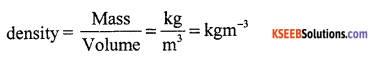(c) What are the fundamental particles of atom.
Electron, proton and neutron.

Question 40.
(a) Mention the postulates of Bohr model of an atom?

1. The electron in the hydrogen atom can move around the nucleus in a circular path of fixed radius and energy. These paths are called orbits.
2. The energy of an electron in the orbit does not change with time.
3. The frequency of radiation absorbed (or) emitted when transition ocurs between two stationary states that differ in energy by ΔE is given by4. The angular momentum of an electron in a given stationary state can be expressed as mvr = $$\frac{\mathrm{nh}}{2 \pi}$$

(b). What is the vaiue of Rydberg’s constant.
R = 2.18 x 1O-18 J.

Question 41.
(a) Mention postulates of kinetic molecular theory of gases.

1. All gases are made up of very large numbers of minute particles called molecules.
2. Intermolecular forces of attraction (or) repulsion are negligible.
3. The pressure exerted by a gas is due to the collisions made by the gas molecules on the walls of the container.
4. The average kinetic energy of the molecules is directly proportional to the absolute temperature.
5. The molecules are involved in rapid, random movement. During their motion, they collide with each other and also against the walls of the container.

OR (Internal choice)

(a) What is an ideal gas? Derive ideal gas equation?
Ideal gas which obey’s Boyle’s law and Charle’s law at all temperatures or PV = RT
Derivation
$$\left(\mathrm{v} \propto \frac{1}{\mathrm{P}}\right) \mathrm{V} \propto \mathrm{T}$$
= V ∝ $$\frac{1}{\mathrm{P}}$$ × P
= V = $$\frac{\mathrm{RT}}{\mathrm{P}}$$ (R = gas constant)
PV = RT or
PV = nRT

(b) Explain surface tension.
Surface tension = $$\frac{\text { Force }}{\text { Area }}$$
The molecules in liquid state on surface experience a net downward force and have more energy than the molecules in bulk, which don’t experience any net force.
If the surface of the liquid is increased by pulling a molecule from the bulk.Question 42.
(a) State Charle’s law.
Charles law : states that volume of a given mass of a gas is directly proportional to temperature at constant pressure.
V ∝ T at constant pressure.

(b) Write Van der Waal’s equation.
Van der Waal’s equation
$$\left(P+\frac{a n^{2}}{V^{2}}\right)(V-n b)=n R T$$

(c) State first law of thermodynamics and write its mathematical statement.
First law of thermodynamics “The energy of an isolated system is constant”.
∆U = q + w

Question 43.
(a) Calculate AG° for conversion of oxygen to ozone; $$\frac{3}{2}$$ O2(g) → O2(g) at 298 K,
if Kp = 2.47 × 10-29.
ΔG° = -2.303RT log Kp
= -2.303 × 8.314 × 298 log 2.47 × 10-29.
= 1.63 kJ/mole

(b) How is ΔU measured calorimetrically.
The heat of combustion of a substance at constant volume is measured by calorimetric method.
The bomb is a closed container made of a heavy steel. The bomb is coated inside with gold to prevent oxidation of steel during combustion reaction. A known mass of the substance whose heat of combustion is to be determined is taken in the platinum cup. The bomb is filled with oxygen at a pressure of 20-25 atm. The bomb is closed with a tight screw cap.

The bomb is surrounded by water bath taken in a insulated outer vessel. The initial temperature of water bath is noted. Let it be t1°C. The combustion is initiated by passing electric current through platinum filament. The heat evolved during combustion raises the temperature of water outside the bomb calorimeter. The highest temperature recorded by thermometer is noted. Let it be t2°C.

Calculation

1. Let the mass of the substance taken be mg.
2. Let the molecular mass of the susbtance taken be ‘M’
3. Let the heat capacity of calorimeter be Q.
4. Let Δt be the rise in temperature.
5. Then Δu, the heat of combustion at constant volume.

Calculated by using the equation
$$\Delta \mathrm{U}=\mathrm{Q} \times \Delta \mathrm{t} \frac{\mathrm{M}}{\mathrm{m}} \mathrm{J}$$

Question 44.
(a) What is buffer solution?
The property of certain solution which resist the change in pH on addition of small amount of acid (or) alkali to it. .

(b) State Lechatelier principle.
If a system in equilibrium is subjected to a change of concentration, temperature (or) pressure, the equilibrium shifts in a direction so as to undo the effect of the change imposed.

(c) Derive PH + POH = 14
Kw = [H3O+][OH] = 10-14
Taking log of minus sign
-log Kw = – log(H3O+) (OH)
-log Kw = – log[H3O+] – log [OH]
PKw = PH + POH = 14
pH + pOH = 14Question 45.
(a) Define ionic product of water.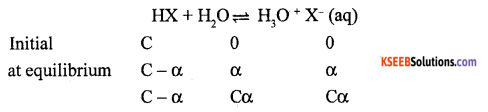$$\mathrm{K}_{\mathrm{a}}=\frac{\left(\mathrm{H}_{3} \mathrm{O}^{+}\right)\left(\mathrm{X}^{-}\right)}{(\mathrm{HX})}=\frac{\mathrm{C} \alpha \cdot \mathrm{C} \alpha}{\mathrm{C}(1-\alpha)}$$
$$\mathrm{K}_{\mathrm{a}}=\frac{\mathrm{C} \alpha^{2}}{1-\alpha}$$